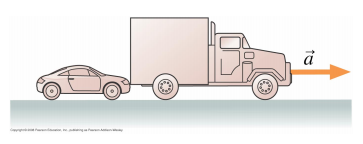# Problem: A small car is pushing a larger truck that has a dead battery. The mass of the truck is larger than the mass of the car. Which of the following statements is true?(a) The car exerts a force on the truck, but the truck doesn’t exert a force on the car.(b) The car exerts a larger force on the truck than the truck exerts on the car. (c) The car exerts the same amount of force on the truck as the truck exerts on the car (d) The truck exerts a larger force on the car than the car exerts on the truck (e) The truck exerts a force on the car, but the car doesn’t exert a force on the truck The correct answer is C. Please explain how you arrived at your answer.

###### FREE Expert Solution

According to Newton's Third law of motion, all forces in the universe occur in equal, but oppositely directed pairs.

87% (376 ratings)###### Problem Details

A small car is pushing a larger truck that has a dead battery. The mass of the truck is larger than the mass of the car. Which of the following statements is true?(a) The car exerts a force on the truck, but the truck doesn’t exert a force on the car.

(b) The car exerts a larger force on the truck than the truck exerts on the car.

(c) The car exerts the same amount of force on the truck as the truck exerts on the car

(d) The truck exerts a larger force on the car than the car exerts on the truck

(e) The truck exerts a force on the car, but the car doesn’t exert a force on the truck